If you find that a pattern or garment does not draft, there is likely an error with your measurements. Sometimes the system will actually show you an error message that corresponds with a measurement.

We will go through a simple draft and show you where the measurements are used. This may help you identify which of your measurements may have an incorrect value. You may find it helpful to open your measurement set in another browser window to review your measurements and also take a look at the illustration showing how the measurement was to be taken. Those illustrations will line up well with the pattern drafting process below.

This draft uses measurements from the Detailed Measurements set. If you want to verify your measurements for a Basic set, please visit the Drafting Errors - Basic Measurements page.

The draft is for a front pattern, but the process is nearly identical for the back pattern using the measurements from the back.

### Center Waist Point

The first point placed is at the center waist.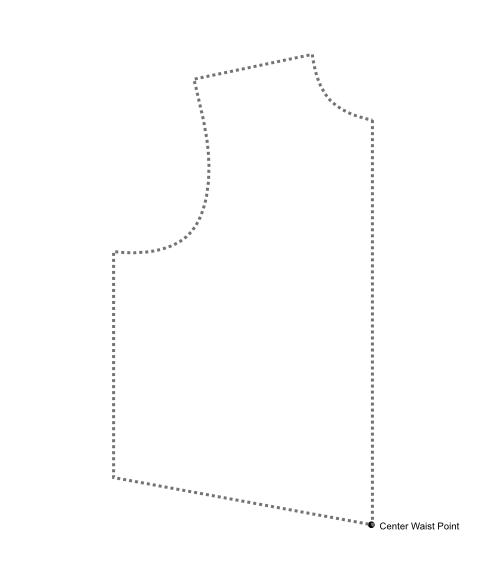### Full Length Point

The next point is placed vertically using the Full Length measurement. There is a horizontal reference line drawn from here.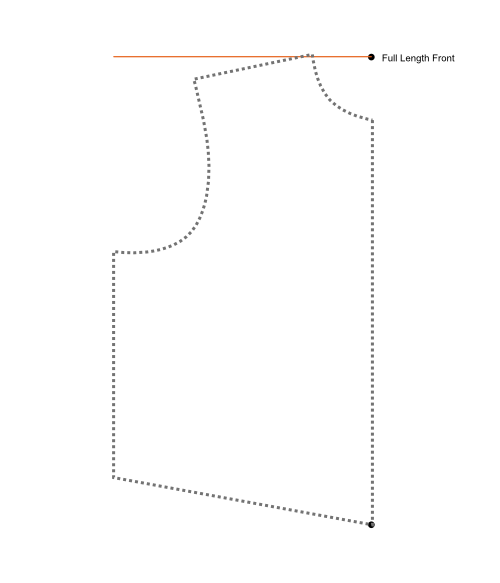### Mid-Arm Width

The next point is placed at the Mid-Arm Width from center. Then a vertical reference line is drawn.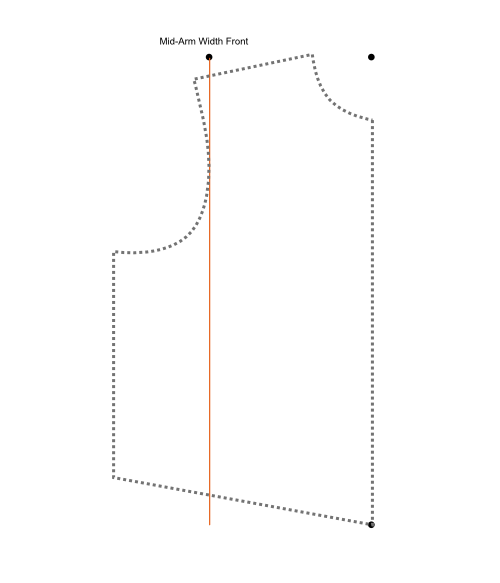### Shoulder Across

The next point is placed at the Shoulder Across measurement from center. Then a vertical reference line is drawn.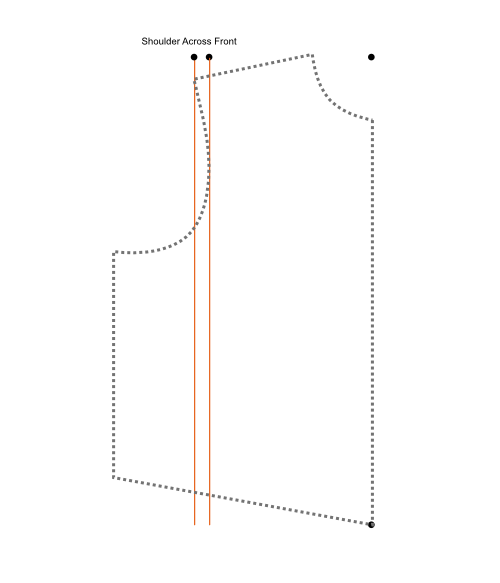### Bust / Chest Width

The next point is placed at the Bust / Chest Width from center. Then a vertical reference line is drawn.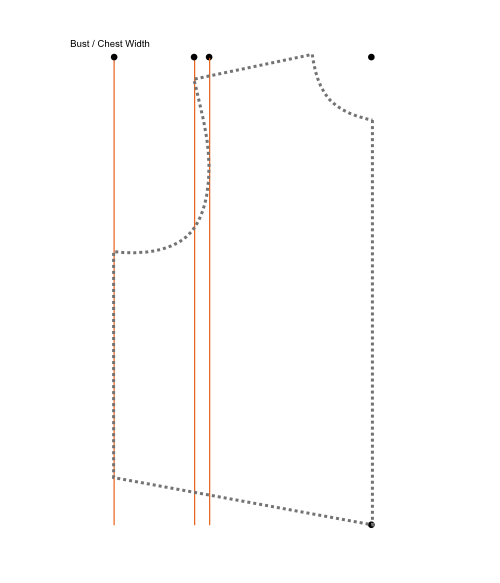### Center Length

The next point is the Center Length measurement up from the waist point.

### Shoulder Slope

The next point is the Shoulder Point. This point is found using a circle. The circle (shown in purple) is drawn with the center on the center waist point. The radius of the circle (shown as red arrowed line) is the Shoulder Sloper length. Where this circle intersects the Shoulder Across reference line is the Shoulder Point. If you get an intersection error that refers to the shoulder slope, that means that the circle does not intersect the line and you should double check your shoulder slope measurement.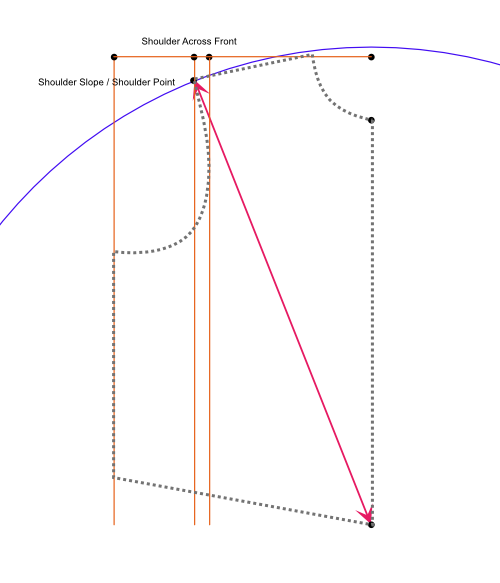### Shoulder Length

The next point is the Side Neck point. This point is found using a circle. The circle (shown in purple) is drawn with the center on the shoulder point. The radius of the circle (shown as red arrowed line) is the Shoulder Length measurement. Where this circle intersects the Full Length reference line is the Side Neck point. If you get an intersection error that refers to the side neck, that means that the circle does not intersect the line and you should double check all the measurements involved.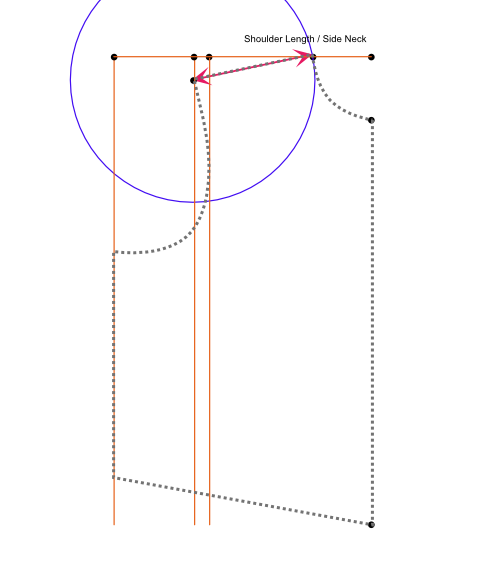### Shoulder to Mid-Arm

The next point is the Mid-Arm point. This point is found using a circle. The circle (shown in purple) is drawn with the center on the shoulder point. The radius of the circle (shown as red arrowed line) is the Shoulder to Mid-Arm measurement. Where this circle intersects the Mid-Arm Width reference line is the Mid-Arm point. If you get an intersection error that refers to the mid-arm, that means that the circle does not intersect the line and you should double check all the measurements involved.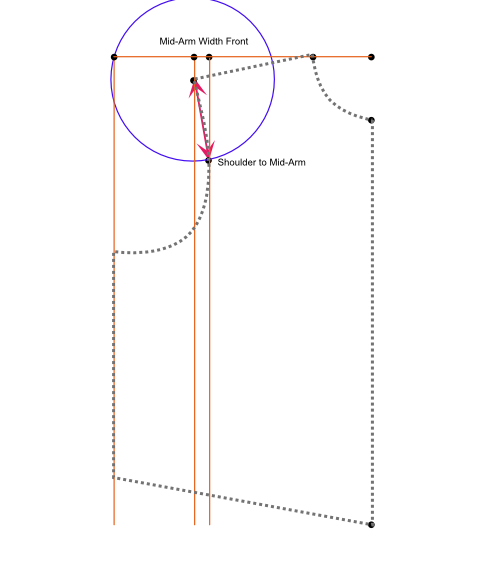### Side Waist Point

The next point is the Side Waist point. This point is found using a circle. The circle (shown in purple) is drawn with the center on the mid-arm point. The radius of the circle (shown as red arrowed line) is the Mid-Armhole Height measurement. Where this circle intersects the Bust / Chest Width reference line is the Side Waist point. If you get an intersection error that refers to the side waist, that means that the circle does not intersect the line and you should double check all the measurements involved.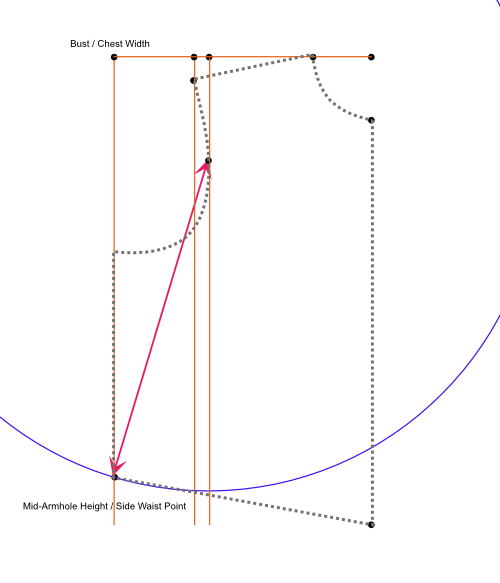### Armpit Point

The next point is the Armpit point. This point is found using a circle. The circle (shown in purple) is drawn with the center on the side waist point. The radius of the circle (shown as red arrowed line) is the Side Length measurement. Where this circle intersects the Bust / Chest Width reference line is the Armpit point. If you get an intersection error that refers to the armpit, that means that the circle does not intersect the line and you should double check all the measurements involved.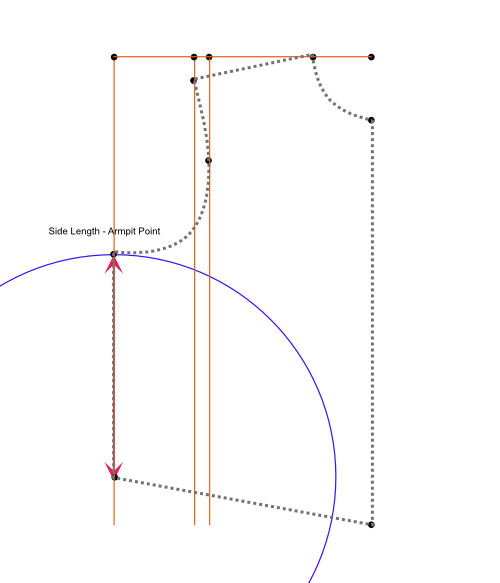Those are the basic points created for patterns / garments using the Detailed Measurement Set. This serves to illustrate where the measurements are used and how errors can occur if those measurements don't line up.